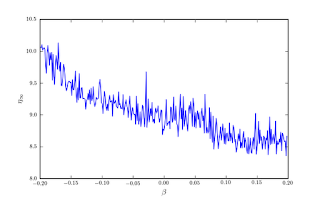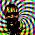## Monday, July 23, 2012

### Convergence for Falkner-Skan Solutions

About 6 months ago Dan Hughes sent me a link to an interesting paper on "chaotic" behavior in the trajectory of iterates in a numerical Falkner-Skan solution. It struck me that the novel results reported in that paper were an artifact of the numerical method, and had little to do with any "chaotic" physics that might be going on in boundary layers or other systems that might be well described by this equation. This is similar to the point I made in the Fun with Filip post: the choice of numerical method matters. Do not rush to judgment about problems until you have brought the most appropriate methods to bear.

There are some things about the paper that are not novel, and others that seem to be nonsense. It is well-known that there can be multiple solutions at given parameter values (non-uniqueness) for this equation, see White. There is the odd claim that "the ﬂow starts to create shock waves in the medium [above the critical wedge angle], which is a representation of chaotic behavior in the ﬂow ﬁeld." Weak solutions (solutions with discontinuities/shocks) and chaotic dynamics are two different things. They use the fact that the method they choose does not converge when two solutions are possible as evidence of chaotic dynamics. Perhaps the iterates really do exhibit chaos, but this is purely an artifact of the method (i.e. there is no physical time in this problem, only the pseudo-time of the iterative scheme). By using a different approach you will get different "dynamics", and with proper choice of method, can get convergence (spectral even!) to any of the multiple solutions depending on what initial condition you give your iterative scheme. They introduce a parameter, $$\eta_{\infty}$$, for the finite value of the independent variable at "infinity" (i.e. the domain is truncated). There is nothing wrong with this (actually it's a commonly used approach for this problem), but it is not a good idea to solve for this parameter as well as the shear at the wall in your Newton iteration. A more careful approach of mapping the boundary point "to infinity" as the grid resolution is increased (following one of Boyd's suggested mappings) removes the need to solve for this parameter, and gives spectral convergence for this problem even in the presence of non-uniqueness and the not uncommon vexation of a boundary condition defined at infinity (all of external aerodynamics has this helpful feature).

Here are some gists for defining and solving the problem using an iterative marching (IVP-style) approach:
• Definition of the third-order nonlinear ODE as a first order system in a Maxima script; this generates fortran90 that I compile into a python module using f2py.
 $\frac{d}{d\phantom{\rule{0.3em}{0ex}}\xi }\phantom{\rule{0.3em}{0ex}}f=\eta \phantom{\rule{0.3em}{0ex}}u$ (1)
 $\frac{d}{d\phantom{\rule{0.3em}{0ex}}\xi }\phantom{\rule{0.3em}{0ex}}u=\eta \phantom{\rule{0.3em}{0ex}}v$ (2)
 $\frac{d}{d\phantom{\rule{0.3em}{0ex}}\xi }\phantom{\rule{0.3em}{0ex}}v=-{\beta }_{0}\phantom{\rule{0.3em}{0ex}}\eta \phantom{\rule{0.3em}{0ex}}f\phantom{\rule{0.3em}{0ex}}v+\beta \phantom{\rule{0.3em}{0ex}}\eta \phantom{\rule{0.3em}{0ex}}{u}^{2}-\beta \phantom{\rule{0.3em}{0ex}}\eta$ (3)
The troublesome BC at infinity:
 $\frac{d}{d\phantom{\rule{0.3em}{0ex}}\xi }\phantom{\rule{0.3em}{0ex}}f=\eta$ (4)
At the wall $$f=0$$ and:  $\frac{{d}^{2}}{d\phantom{\rule{0.3em}{0ex}}{\xi }^{2}}\phantom{\rule{0.3em}{0ex}}f=\alpha \phantom{\rule{0.3em}{0ex}}{\eta }^{2}$ (6)
• Doing a parameter sweep; this method solves for $$\eta_{\infty}$$ and $$\alpha$$; the "converged" $$\eta_{\infty}$$ is a numerical artifact, it is not evidence of anything."Converged" values of domain truncation
• Hyperbolic tangent grid mapping
• Square-root mapping
• ; this one gives decreasing spacing of the first grid point off the wall, and the free-stream boundary point approaches infinity faster than the hyperbolic tangent mapping with increasing number of grid points.First point off-the-wall spacingSpectral Convergence w/square-root mapping
• Script showing convergence to different solutions depending on initialization (non-uniquness); no chaos

I am pretty happy with how well the mapping (grid transformation) with a singularity takes care of the BC defined at infinity. I think the next thing is to try a "jury" (BVP-style) method as an alternative to these marching solutions.

#### 1 comment:

1.Martin Hegedus has an example case that shows shocks/boundary layer separation and non-uniqueness (but not chaos; they converge to steady state solutions).